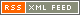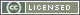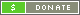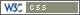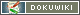## LPRO disciplining with Thunderbolt

From the Time Nuts Mailing List:

In response to request on how I set up my Tbolt with a long time constant to directly discipline a LPRO 101 Rubidium;

I use a standard LPRO 101 Rb as an external replacement for the Tbolt's internal OCXO, and connect the LPRO's external C_Field adjustment input to the Tbolt's Dac out thru a 1K ohm resistor (added as protection for the Tbolt.)

Due to Tbolt's software limitations, an Extended_TC setting method needs to be used for setting Time Constants above 1000 sec.

To calculate the Tbolt's Effective TC setting using this extended TC setting method:

1. The Effective_TC of the TBolt is Actual_TC setting times the Multiplier_Factor
2. The Effective_Damping factor of a Tbolt is Actual_Damping setting divided by the square root of the Multiplier_Factor
3. The Multiplier_Factor is equal to “Dac_Gain used / Actual_EFC_Gain”

Example for setting Tbolt's Effective_TC = 20,000 sec, Effective_Damping factor = 0.6 for a LPRO.

1. Use Multiplier_Factor = 100
2. Dac Gain = +0.90 Hz/V
3. TC = 200 sec
4. Damping = 6.0
5. Set Initial Dac value to +2.5V,

After adjusting the LPRO's to 10 MHz using it's internal freq C field pot (with the external C_Field input floating at 2.5V), it is a good idea to limit the Tbolt's Dac output swing by setting the Max_Dac out to + 3.0 V, and the Min_Dac out to + 2.0 V.

Example of setting Tbolt's Effective_TC = 5,000 sec, Effective_Damping factor = 0.7

(I use this setting to discipline a very stable external dual oven HP 10811.)

1. Use Multiplier factor = 10
2. Dac Gain = -3.24 Hz/V (Actual Gain for this 10811 is -0.324 Hz/V)
3. TC = 500 sec
4. Damping = 2.2

The effective TC can be measured by how long of time that it takes for a Freq offset error to return to zero, best measured by using LH's Dac voltage plot.

The effective Damping can be calculated by how much Freq error overshoots there is, which in turn determines how long of time the Phase error takes to return to zero.

For a damping = 0.6 the Freq overshoot is 33% and Phase correction time is about 3.5 times the Freq correction time (10% phase overshoot)

Using a damping of 0.7, the Freq overshoot is 25%, & phase correction time is 5x the TC setting. (no phase overshoot)

With a damping factor of 1.0, there will be very little Freq overshoot, but that causes the phase correction time to be very long. (overdamped Phase response)

Using Lady Heather, the effective TC and Damping_Factor settings can be verified by plotting the offset freq recovery time to an intentional Dac step error:

1. Disable the control loop (D D)
2. Change the Dac voltage (D S … ) by an amount equal to 1e-10 or 1e-9 (verify on Osc plot)
3. Enable the control loop (D E)
4. Plot the Phase, Dac_Voltage and Osc_Freq over at least 5 times the effective TC setting.

Before replacing a Tbolt's internal Osc with a cheap rubidium, one should understand the noise tradeoffs, especially at low taus. Lots of plots available on request

ws